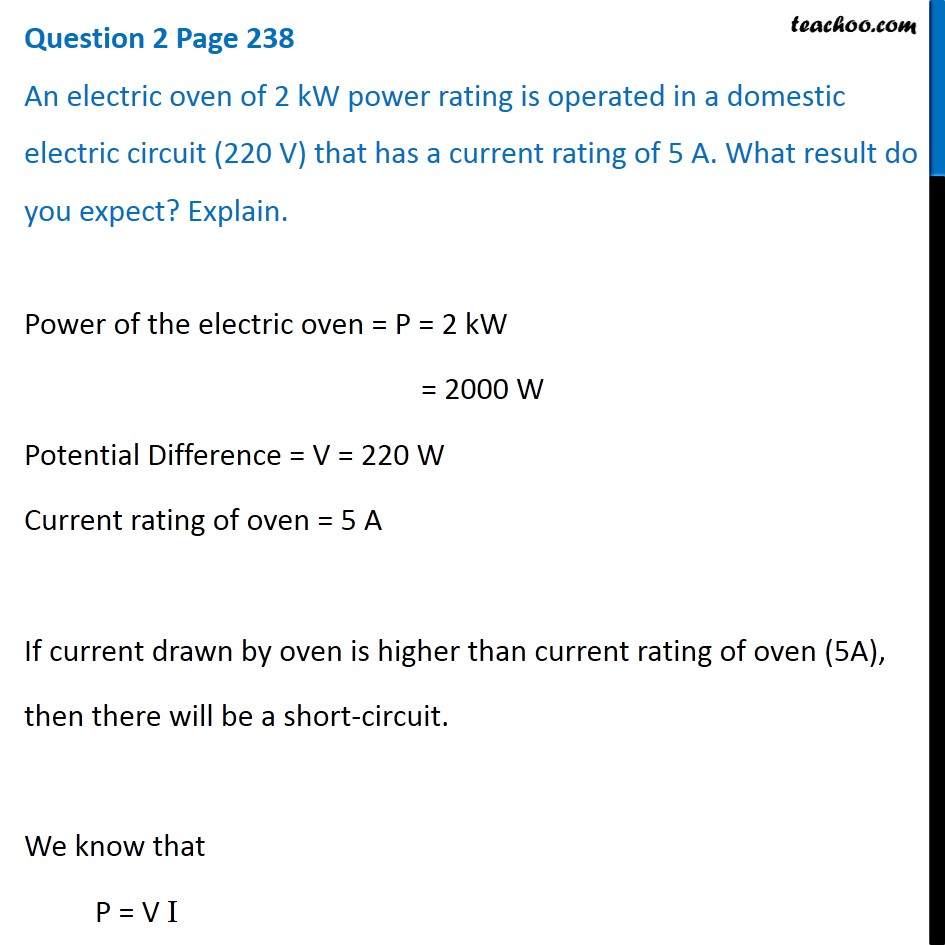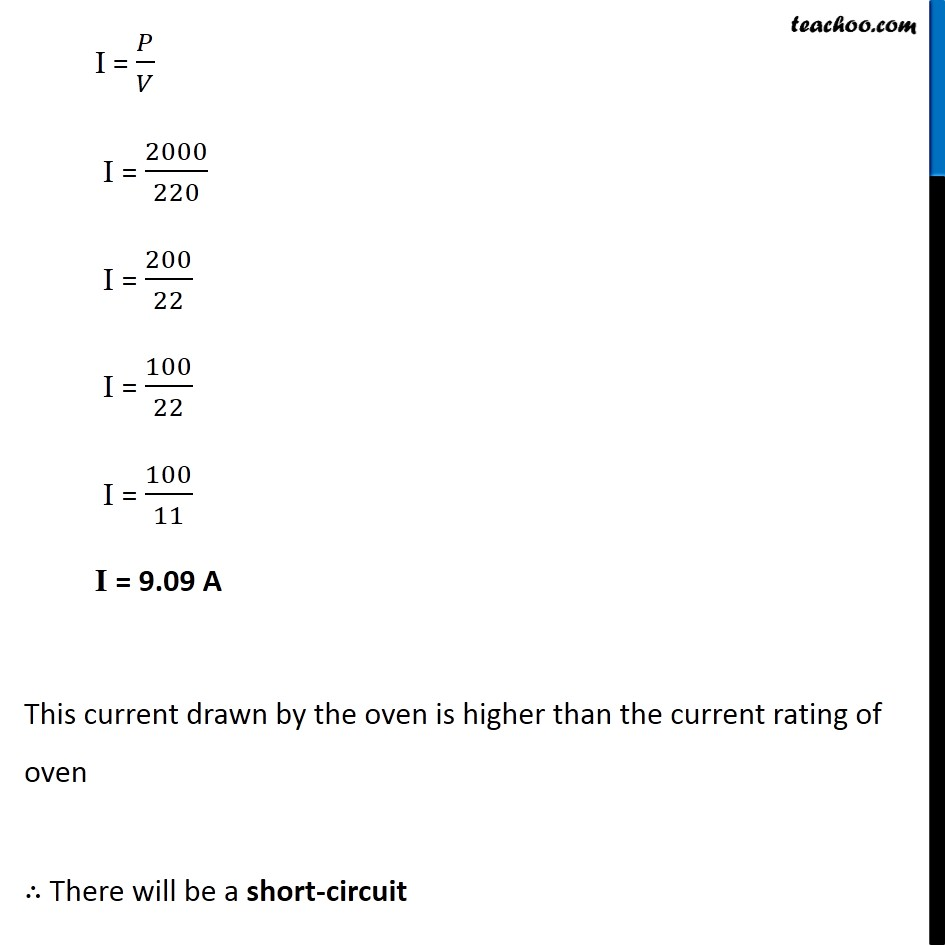Questions from Inside the chapter

Class 10
Chapter 13 Class 10 - Magnetic Effects of Electric Current

## An electric oven of 2 kW power rating is operated in a domestic electric circuit (220 V) that has a current rating of 5 A. What result do you expect? Explain.

####googletag.cmd.push(function() { googletag.display('div-gpt-ad-1669298377854-0'); });(adsbygoogle = window.adsbygoogle || []).push({});

Learn in your speed, with individual attention - Teachoo Maths 1-on-1 Class

### Transcript

Question 2 Page 238 An electric oven of 2 kW power rating is operated in a domestic electric circuit (220 V) that has a current rating of 5 A. What result do you expect? Explain. Power of the electric oven = P = 2 kW = 2000 W Potential Difference = V = 220 W Current rating of oven = 5 A If current drawn by oven is higher than current rating of oven (5A), then there will be a short-circuit. We know that P = V I I = 𝑃/𝑉 I = 2000/220 I = 200/22 I = 100/22 I = 100/11 I = 9.09 A This current drawn by the oven is higher than the current rating of oven ∴ There will be a short-circuit